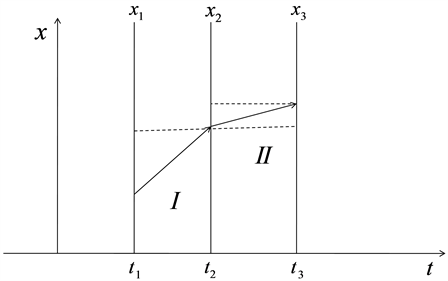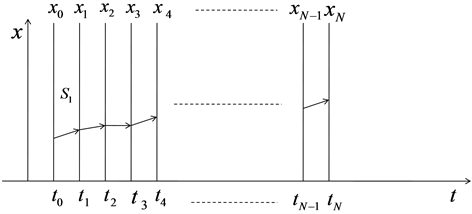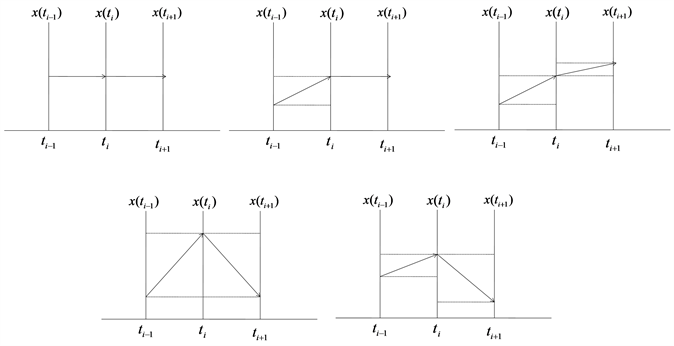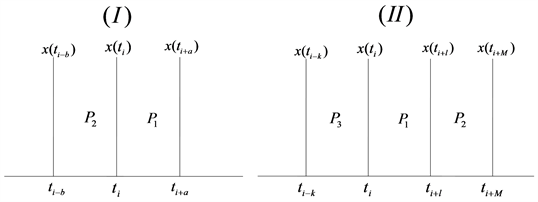﻿ 测度原理下自由粒子的路径积分研究

# 测度原理下自由粒子的路径积分研究Path Integral Study of Free Particles under the Principle of Measurement

Abstract: In this paper, the path integral of free particle motion in quantum mechanics is studied. By introducing the method of measure theory into the description of free particle motion path, therefore, the relationship between the path product components in different measurement sense is constructed.

1. 引言

2. 考虑有限的平面路径积分情形

I区域： $\left\{\begin{array}{c}{t}_{1}\le a\le {t}_{2}\\ {t}_{1}\le a<{t}_{2}\\ {t}_{1}$\left\{\begin{array}{c}{x}_{1}\le b\le {x}_{2}\\ {x}_{1}\le b<{x}_{2}\\ {x}_{1}

II区域： $\left\{\begin{array}{c}{t}_{2}\le a\le {t}_{3}\\ {t}_{2}\le a<{t}_{3}\\ {t}_{2}$\left\{\begin{array}{c}{x}_{2}\le b\le {x}_{3}\\ {x}_{2}\le b<{x}_{3}\\ {x}_{2}Figure 1. Simple plane path integral diagram

$m\left(I\right)=\left({x}_{2}-{x}_{1}\right)\left({t}_{2}-{t}_{1}\right)$

$m\left(II\right)=\left({x}_{3}-{x}_{2}\right)\left({t}_{3}-{t}_{2}\right)$

1) $I+II=I\cup II$，且 $I\cap II=\varnothing$

2) $I+II$ 的测度为 $m\left(I+II\right)=\left({x}_{3}-{x}_{1}\right)\left({t}_{3}-{t}_{1}\right)=m\left(I\right)+m\left(II\right)=\left({x}_{2}-{x}_{1}\right)\left({t}_{2}-{t}_{1}\right)+\left({x}_{3}-{x}_{2}\right)\left({t}_{3}-{t}_{2}\right)$

$U\left(t\right)U\left(x,t;{x}^{\prime },{t}^{\prime }\right)=A\underset{\text{allpath}}{\sum }\mathrm{exp}\left\{is\left[x\left(t\right)\right]/\hslash \right\}$

$U\left(x,t;{x}^{\prime },{t}^{\prime }\right)={A}^{\prime }\mathrm{exp}\left\{i{s}_{cl}/\hslash \right\}$

$\frac{{x}_{cl}\left({t}_{3}\right)-{x}_{2}}{{t}_{3}-{t}_{2}}=\frac{{x}_{1}-{x}_{2}}{{t}_{1}-{t}_{2}}$

$m\left(I\right)=\left({x}_{1}-{x}_{2}\right)\left({t}_{1}-{t}_{2}\right)$

$m\left(II\right)=\left({x}_{cl}\left({t}_{3}\right)-{x}_{2}\right)\left({t}_{3}-{t}_{2}\right)$

$⇒$ $\frac{m\left(I\right)}{{\left({t}_{1}-{t}_{2}\right)}^{2}}=\frac{m\left(II\right)}{{\left({t}_{3}-{t}_{2}\right)}^{2}}$

$⇒$ ${\left({t}_{3}-{t}_{2}\right)}^{2}m\left(I\right)={\left({t}_{1}-{t}_{2}\right)}^{2}m\left( I I \right)$

$v=\frac{{x}_{1}-{x}_{2}}{{t}_{1}-{t}_{2}}=\frac{m\left(I\right)}{{\left({t}_{1}-{t}_{2}\right)}^{2}}=\frac{m\left(II\right)}{{\left({t}_{3}-{t}_{2}\right)}^{2}}$

$⇒$ ${S}_{cl}={\int }_{{t}_{2}}^{{t}_{1}}L\text{d}{t}_{3}=\frac{1}{2}{\int }_{{t}_{2}}^{{t}_{1}}m{v}^{2}\text{d}{t}_{3}={m{t}_{3}\frac{m{\left(I\right)}^{2}}{2{\left({t}_{1}-{t}_{2}\right)}^{4}}|}_{{t}_{2}}^{{t}_{1}}=\frac{1}{2}m\frac{m{\left(I\right)}^{2}}{{\left({t}_{1}-{t}_{2}\right)}^{3}}$

$U\left({x}_{1},{t}_{1};{x}_{2},{t}_{2}\right)={A}^{\prime }\mathrm{exp}\left[\frac{imm{\left(I\right)}^{2}}{2{\left({t}_{1}-{t}_{2}\right)}^{3}}/\hslash \right]$

$U\left({x}_{1},{t}_{1};{x}_{2},0\right)=U\left({x}_{1},{t}_{1};{x}_{2}\right)={\left(\frac{m}{2\pi \hslash it}\right)}^{1/2}\mathrm{exp}\left[\frac{imm{\left(I\right)}^{2}}{2{t}_{1}\hslash }\right]$.

3. 趋近无穷时的平面路径积分情形Figure 2. Multi-planar path integral diagram

$U\left({x}_{N},{t}_{N};{x}_{0},{t}_{0}\right)={\int }_{{x}_{0}}^{{x}_{N}}\mathrm{exp}\left\{is\left[x\left(t\right)\right]/\hslash \right\}D\left[x\left(t\right)\right]$

$m\left(S\right)=\underset{i=0}{\overset{N-1}{\sum }}\frac{\left({x}_{i+1}-{x}_{i}\right)\left({t}_{N}-{t}_{0}\right)}{N}$

$v=\frac{m\left(S\right)}{{\theta }^{2}}$ 代入，就有

$S={\frac{1}{2}m\frac{m{\left(S\right)}^{2}}{{\theta }^{4}}t|}_{{t}_{0}}^{{t}_{N}}⇒S=\frac{mm{\left(S\right)}^{2}N}{2{\theta }^{3}}$

$U\left({x}_{N},{t}_{N};{x}_{0},{t}_{0}\right)={\int }_{{x}_{0}}^{{x}_{N}}\mathrm{exp}\left\{\frac{imm{\left(S\right)}^{2}N}{2{\theta }^{3}\hslash }\right\}D\left[x\left(t\right)\right]$

$U\left({x}_{N},{t}_{N};{x}_{0},{t}_{0}\right)=\underset{\begin{array}{l}N\to \infty \\ \theta \to 0\end{array}}{\mathrm{lim}}A\underset{-\infty }{\overset{\infty }{\int }}\underset{-\infty }{\overset{\infty }{\int }}\cdots \underset{-\infty }{\overset{\infty }{\int }}\mathrm{exp}\left[\frac{im{\left(S\right)}^{2}Nm}{2{\theta }^{3}\hslash }\right]×\text{d}{x}_{1}\cdots \text{d}{x}_{N-1}$

$S=\underset{i=0}{\overset{N-1}{\sum }}\frac{m}{2}{\left(\frac{{x}_{i+1}-{x}_{i}}{\theta }\right)}^{2}\theta$

$⇒$ $U\left({x}_{N},{t}_{N};{x}_{0},{t}_{0}\right)=\underset{\begin{array}{l}N\to \infty \\ \theta \to 0\end{array}}{\mathrm{lim}}A\underset{\left(N-1\right)}{\underset{-\infty }{\overset{\infty }{\int }}}\mathrm{exp}\left[\frac{im}{2\hslash }\underset{i=0}{\overset{N-1}{\sum }}\frac{{\left({x}_{i+1}-{x}_{i}\right)}^{2}}{\theta }\right]×\text{d}{x}_{1}\cdots \text{d}{x}_{N-1}$

$⇒$ $U=\underset{\begin{array}{l}N\to \infty \\ \theta \to 0\end{array}}{\mathrm{lim}}A\underset{\left(N-1\right)}{\underset{-\infty }{\overset{\infty }{\int }}}\mathrm{exp}\left\{i\underset{i=0}{\overset{N-1}{\sum }}\left[{\left(\frac{m}{2\hslash \theta }\right)}^{1/2}{x}_{i+1}-{\left(\frac{m}{2\hslash \theta }\right)}^{1/2}{x}_{i}\right]\right\}$ (1)

$⇒$ ${U}^{\prime }=\underset{N\to \infty }{\mathrm{lim}}{A}^{\prime }\underset{\left(N-1\right)}{\underset{-\infty }{\overset{\infty }{\int }}}\mathrm{exp}\left[i\underset{i=0}{\overset{N-1}{\sum }}{\left({y}_{i+1}-{y}_{i}\right)}^{2}\right]\text{d}{y}_{1}\cdots \text{d}{y}_{N-1}$ (2)

${A}^{\prime }=A{\left(\frac{2\hslash \theta }{m}\right)}^{\left(N-1\right)/2}$

$t=2$ 时，得到

${\int }_{-\infty }^{\infty }\mathrm{exp}\left\{i\left[{\left({y}_{2}-{y}_{1}\right)}^{2}+{\left({y}_{1}-{y}_{0}\right)}^{2}\right]\right\}\text{d}{y}_{1}={\left(\frac{i\pi }{2}\right)}^{1/2}{\text{e}}^{-{\left({y}_{2}-{y}_{0}\right)}^{2}/2i}$

$t=3$ 时，得到

${\left(\frac{i\pi }{2}\right)}^{1/2}{\int }_{-\infty }^{\infty }{\text{e}}^{-{\left({y}_{3}-{y}_{2}\right)}^{2}/i}{\text{e}}^{-{\left({y}_{2}-{y}_{0}\right)}^{2}/2i}\text{d}{y}_{2}=\frac{i\pi }{3}{\text{e}}^{-{\left({y}_{3}-{y}_{0}\right)}^{2}/3i}$

$t=N$ 时，得到

${\left(\frac{i\pi }{2}\right)}^{\left(N-2\right)/2}{\int }_{-\infty }^{\infty }{\text{e}}^{-{\left({y}_{N}-{y}_{N-1}\right)}^{2}/i}{\text{e}}^{-{\left({y}_{N-1}-{y}_{0}\right)}^{2}/\left(N-1\right)i}\text{d}{y}_{N-1}=\frac{{\left(i\pi \right)}^{\left(N-1\right)/2}}{{N}^{1/2}}{\text{e}}^{-{\left({y}_{N}-{y}_{0}\right)}^{2}/Ni}$

$\left\{\begin{array}{l}\frac{{\left(i\pi \right)}^{\left(N-1\right)/2}}{{N}^{1/2}}{\text{e}}^{-{\left({y}_{N}-{y}_{0}\right)}^{2}/Ni}\\ {y}_{N}={\left(\frac{m}{2\hslash \theta }\right)}^{1/2}{x}_{N},{y}_{0}={\left(\frac{m}{2\hslash \theta }\right)}^{1/2}{x}_{0}\end{array}$

$⇒$ $\frac{{\left(i\pi \right)}^{\left(N-1\right)/2}}{{N}^{1/2}}{\text{e}}^{-\left[{\left(\frac{m}{2\hslash \theta }\right)}^{1/2}{x}_{N}-{\left(\frac{m}{2\hslash \theta }\right)}^{1/2}{x}_{0}\right]/Ni}=\frac{{\left(i\pi \right)}^{\left(N-1\right)/2}}{{N}^{1/2}}{\text{e}}^{-m{\left({x}_{N}-{x}_{0}\right)}^{2}/2\hslash \theta Ni}$

$U=A{\left(\frac{2\pi \hslash \theta i}{m}\right)}^{N/2}{\left(\frac{m}{2\pi \hslash \theta iN}\right)}^{1/2}\mathrm{exp}\left[\frac{im{\left({x}_{N}-{x}_{0}\right)}^{2}}{2\hslash N\theta }\right]$

$U\left({x}_{N},{t}_{N};{x}_{0},{t}_{0}\right)=\underset{\begin{array}{l}N\to \infty \\ \theta \to 0\end{array}}{\mathrm{lim}}{\left(\frac{2\pi \hslash \theta i}{m}\right)}^{-N/2}\underset{\left(N-1\right)}{\underset{-\infty }{\overset{\infty }{\int }}}\mathrm{exp}\left[\frac{imm{\left(s\right)}^{2}N}{2\hslash {\theta }^{3}}\right]×\text{d}{x}_{1}\cdots \text{d}{x}_{N-1}$Figure 3. Several adjacent rectangular graphs

${\mu }^{\ast }\left(A△B\right)={\mu }^{\ast }\left(A\cup B-\left\{\left(x,y\right)|x={t}_{i},x\left({t}_{i-1}\right)\le y\le x\left({t}_{i}\right)\right\}\right)$

$m\left(A\cup B\right)=m\left(A\right)+m\left(B\right)=\sum \frac{\left(x\left({t}_{i}\right)-x\left({t}_{i-p}\right)\right)\left({t}_{i}-{t}_{i-p}\right)}{p}+\sum \frac{\left(x\left({t}_{i+q}\right)-x\left({t}_{i}\right)\right)\left({t}_{i+q}-{t}_{i}\right)}{q}<\epsilon$

${U}^{\ast }\left({x}_{N},{t}_{N};{x}_{0},{t}_{0}\right)=\underset{\begin{array}{l}N\to \infty \\ \theta \to 0\end{array}}{\mathrm{lim}}\underset{\left(N-1\right)}{\underset{-\infty }{\overset{\infty }{\int }}}{\left(\frac{2\pi \hslash \theta i}{m}\right)}^{-N/2}\mathrm{exp}\left[\frac{im{\mu }^{\ast }{\left(A△B\right)}^{2}N}{2\hslash {\theta }^{3}}\right]×\text{d}{x}_{1}\cdots \text{d}{x}_{N-1}$

$\begin{array}{l}{U}^{\ast }\left({x}_{N},{t}_{N};{x}_{0},{t}_{0}\right)\\ \le \underset{\begin{array}{l}N\to \infty \\ \theta \to 0\end{array}}{\mathrm{lim}}{\left(\frac{2\pi \hslash \theta i}{m}\right)}^{-N/2}\underset{\left(N-1\right)}{\underset{-\infty }{\overset{\infty }{\int }}}\mathrm{exp}\left[\frac{im\left({\mu }^{\ast }{\left(A\right)}^{2}+2{\mu }^{\ast }\left(A\right){\mu }^{\ast }\left(B\right)+{\mu }^{\ast }{\left(B\right)}^{2}\right)N}{2\hslash {\theta }^{3}}\right]×\text{d}{x}_{1}\cdots \text{d}{x}_{N-1}\\ \le \underset{\begin{array}{l}N\to \infty \\ \theta \to 0\end{array}}{\mathrm{lim}}{\left(\frac{2\pi \hslash \theta i}{m}\right)}^{-N/2}\underset{\left(N-1\right)}{\underset{-\infty }{\overset{\infty }{\int }}}\mathrm{exp}\left[\frac{im\left(2{\mu }^{\ast }{\left(A\right)}^{2}+2{\mu }^{\ast }{\left(B\right)}^{2}\right)N}{2\hslash {\theta }^{3}}\right]×\text{d}{x}_{1}\cdots \text{d}{x}_{N-1}\\ =\underset{\begin{array}{l}N\to \infty \\ \theta \to 0\end{array}}{\mathrm{lim}}{\left(\frac{2\pi \hslash \theta i}{m}\right)}^{-N/2}\underset{\left(N-1\right)}{\underset{-\infty }{\overset{\infty }{\int }}}\mathrm{exp}\left[\frac{2im{\left(\underset{A,B\subset S}{\mathrm{inf}}m\left(S\right)\right)}^{2}N}{\hslash {\theta }^{3}}\right]×\text{d}{x}_{1}\cdots \text{d}{x}_{N-1}\\ <\underset{\begin{array}{l}N\to \infty \\ \theta \to 0\end{array}}{\mathrm{lim}}{\left(\frac{2\pi \hslash \theta i}{m}\right)}^{-N/2}\underset{\left(N-1\right)}{\underset{-\infty }{\overset{\infty }{\int }}}\mathrm{exp}\left[\frac{imN{\epsilon }^{2}}{2\hslash {\theta }^{3}}\right]×\text{d}{x}_{1}\cdots \text{d}{x}_{N-1}\end{array}$

${U}_{L}\left({x}_{N},{t}_{N};{x}_{0},{t}_{0}\right)=\underset{\begin{array}{l}N\to \infty \\ \theta \to 0\end{array}}{\mathrm{lim}}{\left(\frac{2\pi \hslash \theta i}{m}\right)}^{-N/2}\underset{\left(N-1\right)}{\underset{-\infty }{\overset{\infty }{\int }}}\mathrm{exp}\left[\frac{im\mu {\left(A\right)}^{2}N}{2\hslash {\theta }^{3}}\right]×\text{d}{x}_{1}\cdots \text{d}{x}_{N-1}$

${U}_{\epsilon }\left({x}_{N},{t}_{N};{x}_{0},{t}_{0}\right)=\underset{\begin{array}{l}N\to \infty \\ \theta \to 0\end{array}}{\mathrm{lim}}{\left(\frac{2\pi \hslash \theta i}{m}\right)}^{-N/2}\underset{\left(N-1\right)}{\underset{-\infty }{\overset{\infty }{\int }}}\mathrm{exp}\left[\frac{im{\epsilon }^{2}N}{2\hslash {\theta }^{3}}\right]×\text{d}{x}_{1}\cdots \text{d}{x}_{N-1}$

${U}_{A}\left({x}_{N},{t}_{N};{x}_{0},{t}_{0}\right)=\underset{\begin{array}{l}N\to \infty \\ \theta \to 0\end{array}}{\mathrm{lim}}{\left(\frac{2\pi \hslash \theta i}{m}\right)}^{-N/4}\underset{\left(N-1\right)}{\underset{-\infty }{\overset{\infty }{\int }}}\mathrm{exp}\left[\frac{im{\mu }^{\ast }{\left(A\right)}^{2}N}{4\hslash {\theta }^{3}}\right]×\text{d}{x}_{1}\cdots \text{d}{x}_{N-1}$

${U}_{B}\left({x}_{N},{t}_{N};{x}_{0},{t}_{0}\right)=\underset{\begin{array}{l}N\to \infty \\ \theta \to 0\end{array}}{\mathrm{lim}}{\left(\frac{2\pi \hslash \theta i}{m}\right)}^{-N/4}\underset{\left(N-1\right)}{\underset{-\infty }{\overset{\infty }{\int }}}\mathrm{exp}\left[\frac{im{\mu }^{\ast }{\left(B\right)}^{2}N}{4\hslash {\theta }^{3}}\right]×\text{d}{x}_{1}\cdots \text{d}{x}_{N-1}$

$A-C={S}_{1}\cup {S}_{2}-\left({t}_{a},x\left({t}_{a}\right)\right)-\left({t}_{a+d},x\left({t}_{a+d}\right)\right)⇒A-C\subset {S}_{1}\cup {S}_{2}$

$m\left(A-C\right)\le \underset{i=1,2}{\sum }m\left({S}_{i}\right)=\underset{p=0}{\overset{j-1}{\sum }}\frac{\left(x\left({t}_{a-p}\right)-x\left({t}_{a-p-1}\right)\right)\left({t}_{a}-{t}_{a-j}\right)}{j}+\underset{q=0}{\overset{f-d-1}{\sum }}\frac{\left(x\left({t}_{a+d+q+1}\right)-x\left({t}_{a+d+q}\right)\right)\left({t}_{a+d+q+1}-{t}_{a+d+q}\right)}{f-d}$

$A-C=\left\{\begin{array}{l}{S}_{1}\cup {S}_{2}-\left({t}_{a},x\left({t}_{a}\right)\right)-\left({t}_{a+d},x\left({t}_{a+d}\right)\right)\\ {S}_{1}\cup {S}_{2}-\left\{\left(x,y\right)|x={t}_{a},x\left({t}_{a-1}\right)\le y\le x\left({t}_{a}\right)\right\}-\left\{\left(x,y\right)|x={t}_{a+d},x\left({t}_{a+d}\right)\le y\le x\left({t}_{a+d+1}\right)\right\}\end{array}$,

$\xi =\underset{p=0}{\overset{j-1}{\sum }}\frac{\left(x\left({t}_{a-p}\right)-x\left({t}_{a-p-1}\right)\right)\left({t}_{a}-{t}_{a-j}\right)}{j}+\underset{q=0}{\overset{f-d-1}{\sum }}\frac{\left(x\left({t}_{a+d+q+1}\right)-x\left({t}_{a+d+q}\right)\right)\left({t}_{a+d+q+1}-{t}_{a+d+q}\right)}{f-d}$

${U}_{J}\left({x}_{N},{t}_{N};{x}_{0},{t}_{0}\right)=\underset{\begin{array}{l}N\to \infty \\ \theta \to 0\end{array}}{\mathrm{lim}}{\left(\frac{2\pi \hslash \theta i}{m}\right)}^{-N/2}\underset{\left(N-1\right)}{\underset{-\infty }{\overset{\infty }{\int }}}\mathrm{exp}\left[\frac{im\mu {\left(B\right)}^{2}N}{2\hslash {\theta }^{3}}\right]×\text{d}{x}_{1}\cdots \text{d}{x}_{N-1}$.

$m\left(P\right)=m\left(P\cap {P}_{1}\right)+m\left(P-{P}_{1}\right)\le m\left({P}_{1}\right)+m\left( P 2 \right)$Figure 4. Arbitrary path integral diagram

$m\left({P}_{1}\right)=\underset{k=1}{\overset{a}{\sum }}\frac{\left({t}_{i+k}-{t}_{i+k-1}\right)\left(x\left({t}_{i+k}\right)-x\left({t}_{i+k-1}\right)\right)}{a}$

$m\left({P}_{2}\right)=\underset{k=1}{\overset{b}{\sum }}\frac{\left({t}_{i-k+1}-{t}_{i-k}\right)\left(x\left({t}_{i-k+1}\right)-x\left({t}_{i-k}\right)\right)}{b}$

$m\left(P\right)\le \underset{k=1}{\overset{a,b}{\sum }}\left[\frac{\left({t}_{i+k}-{t}_{i+k-1}\right)\left(x\left({t}_{i+k}\right)-x\left({t}_{i+k-1}\right)\right)}{a}+\frac{\left({t}_{i-k+1}-{t}_{i-k}\right)\left(x\left({t}_{i-k+1}\right)-x\left({t}_{i-k}\right)\right)}{b}\right]$

${U}_{P}=\underset{\begin{array}{l}N\to \infty \\ \theta \to 0\end{array}}{\mathrm{lim}}{\left(\frac{2\pi \hslash \theta i}{m}\right)}^{-N/2}\underset{\left(N-1\right)}{\underset{-\infty }{\overset{\infty }{\int }}}\mathrm{exp}\left[\frac{imm{\left(P\right)}^{2}N}{2\hslash {\theta }^{3}}\right]×\text{d}{x}_{1}\cdots \text{d}{x}_{N-1}$

${U}_{P}\le \underset{\begin{array}{l}N\to \infty \\ \theta \to 0\end{array}}{\mathrm{lim}}{\left(\frac{2\pi \hslash \theta i}{m}\right)}^{-N/2}\underset{\left(N-1\right)}{\underset{-\infty }{\overset{\infty }{\int }}}\mathrm{exp}\left[\frac{im{\left(m\left({P}_{1}\right)+m\left({P}_{2}\right)\right)}^{2}N}{2\hslash {\theta }^{3}}\right]×\text{d}{x}_{1}\cdots \text{d}{x}_{N-1}$ (3)

$\left(3\right)\ge \underset{\begin{array}{l}N\to \infty \\ \theta \to 0\end{array}}{\mathrm{lim}}{\left(\frac{2\pi \hslash \theta i}{m}\right)}^{-N/2}\underset{\begin{array}{l}-\infty \\ \left(N-1\right)\end{array}}{\overset{\infty }{\int }}\mathrm{exp}\left[\frac{2imm\left({P}_{1}\right)m\left({P}_{2}\right)N}{\hslash {\theta }^{3}}\right]×\text{d}{x}_{1}\cdots \text{d}{x}_{N-1}$

$P={P}_{1}\cup {P}_{2}\cup {P}_{3},P\subset X,{\mu }^{\ast }\left(P\right)={\mu }^{\ast }\left(P\cap {P}_{1}\right)+{\mu }^{\ast }\left(P-{P}_{1}\right)$

$⇒$ $m\left(P\right)=m\left(P\cap {P}_{1}\right)+m\left(P-{P}_{1}\right)\le m\left({P}_{1}\right)+m\left({P}_{2}\right)+m\left( P 3 \right)$

$m\left({P}_{1}\right)=\underset{s=1}{\overset{L}{\sum }}\frac{\left({t}_{i+s}-{t}_{i+s-1}\right)\left(x\left({t}_{i+s}\right)-x\left({t}_{i+s-1}\right)\right)}{L}$

$m\left({P}_{2}\right)=\underset{s=1}{\overset{M-L}{\sum }}\frac{\left({t}_{i+L+s}-{t}_{i+L+s-1}\right)\left(x\left({t}_{i+L+s}\right)-x\left({t}_{i+L+s-1}\right)\right)}{M-L}$

$m\left({P}_{3}\right)=\underset{s=1}{\overset{K}{\sum }}\frac{\left({t}_{i-s+1}-{t}_{i-s}\right)\left(x\left({t}_{i-s+1}\right)-x\left({t}_{i-s}\right)\right)}{K}$

$\begin{array}{c}m\left(P\right)\le \underset{s=1}{\overset{L}{\sum }}\frac{\left({t}_{i+s}-{t}_{i+s-1}\right)\left(x\left({t}_{i+s}\right)-x\left({t}_{i+s-1}\right)\right)}{L}\\ \text{\hspace{0.17em}}+\underset{s=1}{\overset{M-L}{\sum }}\frac{\left({t}_{i+L+s}-{t}_{i+L+s-1}\right)\left(x\left({t}_{i+L+s}\right)-x\left({t}_{i+L+s-1}\right)\right)}{M-L}\\ \text{\hspace{0.17em}}+\underset{s=1}{\overset{K}{\sum }}\frac{\left({t}_{i-s+1}-{t}_{i-s}\right)\left(x\left({t}_{i-s+1}\right)-x\left({t}_{i-s}\right)\right)}{K}\end{array}$

$\begin{array}{c}{U}_{P}=\underset{\begin{array}{l}N\to \infty \\ \theta \to 0\end{array}}{\mathrm{lim}}{\left(\frac{2\pi \hslash \theta i}{m}\right)}^{-N/2}\underset{\left(N-1\right)}{\underset{-\infty }{\overset{\infty }{\int }}}\mathrm{exp}\left[\frac{imm{\left(P\right)}^{2}N}{2\hslash {\theta }^{3}}\right]×\text{d}{x}_{1}\cdots \text{d}{x}_{N-1}\\ \le \underset{\begin{array}{l}N\to \infty \\ \theta \to 0\end{array}}{\mathrm{lim}}{\left(\frac{2\pi \hslash \theta i}{m}\right)}^{-N/2}\underset{\left(N-1\right)}{\underset{-\infty }{\overset{\infty }{\int }}}\mathrm{exp}\left[\frac{im{\left(m\left({P}_{1}\right)+m\left({P}_{2}\right)+m\left({P}_{3}\right)\right)}^{2}N}{2\hslash {\theta }^{3}}\right]×\text{d}{x}_{1}\cdots \text{d}{x}_{N-1}\end{array}$ (4)

$\begin{array}{c}\left(4\right)\ge \underset{\begin{array}{l}N\to \infty \\ \theta \to 0\end{array}}{\mathrm{lim}}{\left(\frac{2\pi \hslash \theta i}{m}\right)}^{-N/2}\underset{\begin{array}{l}-\infty \\ \left(N-1\right)\end{array}}{\overset{\infty }{\int }}\mathrm{exp}\left[\frac{4imm\left({P}_{1}\right)\sqrt{m\left({P}_{2}\right)m\left({P}_{3}\right)}N}{\hslash {\theta }^{3}}\right]×\text{d}{x}_{1}\cdots \text{d}{x}_{N-1}\\ =\underset{\begin{array}{l}N\to \infty \\ \theta \to 0\end{array}}{\mathrm{lim}}{\left(\frac{2\pi \hslash \theta i}{m}\right)}^{-N/2}\underset{\left(N-1\right)}{\underset{-\infty }{\overset{\infty }{\int }}}\mathrm{exp}\left[\frac{2imm{\left({P}_{1}\right)}^{2}N}{\hslash {\theta }^{3}}\right]×\text{d}{x}_{1}\cdots \text{d}{x}_{N-1}\end{array}$

$\left\{\begin{array}{l}m\left({P}_{1}\right)=2m\left({P}_{2}\right)=2m\left({P}_{3}\right)\\ m\left(P\right)=2m\left( P 1 \right)\end{array}$

4. 总结

 A. H. 柯尔莫戈洛. 夫函数论与泛函分析初步[M]. 北京: 高等教育出版社, 2006.

 Shankar, R. (2007) Princi-ples of Quantum Mechanics. 2nd Edition, World Book Inc., Beijing.

 塔诺季. 量子力学[M]. 北京: 高等教育出版社, 2014.

 朗道. 量子力学(非相对论理论) [M]. 北京: 高等教育出版社, 2008.

 Halmos, P.R. (2007) Measure Theory. World Book Inc., Beijing.

Top